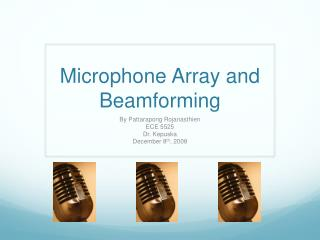# Microphone Array and Beamforming - PowerPoint PPT PresentationDownload PresentationMicrophone Array and Beamforming

Microphone Array and BeamformingDownload Presentation## Microphone Array and Beamforming

- - - - - - - - - - - - - - - - - - - - - - - - - - - E N D - - - - - - - - - - - - - - - - - - - - - - - - - - -
##### Presentation Transcript

1. Microphone Array and Beamforming By Pattarapong Rojanasthien ECE 5525 Dr. Kepuska December 8th, 2008

2. Overview • Introduction • Background • Microphone Arrays • Beamforming • Signal to Noise Ratio • Solution and Approach • Results • Conclusion

3. Introduction • The goals for the project are the following • Learn the basic concept of microphone array • Become familiar and learn how to use a program that perform Beamforming • Show that Beamforming will enhance the signal quality based on SNR

4. Microphone Array • Multiple microphones set up in certain formation depend on the application. • Line • Circle • Rectangle • Each microphone will capture the sound from the source at the different time due to distance from the source. • Therefore, there is a time delay in each signal.

5. Microphone Array

6. Multi-Channel Signal Comparison

7. Time Delay • Note that the distance between another microphone (not the reference) to the source, which denote as g, can be compute with trigonometry cos  = d/g => d = g cos. • The distance from source to Ref Mic: d = 30 in. • The distance from source to Mic x: g = 34.73 in. • The distance between mic: N = 17.5 in. • Sampling Frequency: fs = 22050 samples/sec. • Speed of sound: c = 345 m/sec. • Find d’ = 34.73 – 30 = 4.73. • Find Sample/meter => 22050/345 = 63.913 samples/m. • Turn into inches => 63.913 x 0.0254 = 1.62 • To find time delay, we need distance d’ x = 4.73 x 1.62 = 7.7 samples, round up to be 8 samples.

8. Example of Microphone Array

9. Beamforming • A technique that rearrange the mixture signals from the microphone array, so that the signals from the source that we want are lined up before combine them all up into one signal • Since signals from the specified source are on the same phase, they will add each other up. • The noise signals may either add each other up or cancel each other out. • The general form of the beamforming output based on delay and sum

10. Beamforming Pattern

11. Signal to Noise Ratio • The measurement of the signal quality • Basically, it is the magnitude of • One way to calculate it is to find noise from voice activity detection • Measure the mean of the energy during the last t1 seconds => E1 • Measure the mean of the energy during the last t2 seconds => E2 • Calculate the speech threshold T2 = E2 + Ex • If E1 > Ts, speech is detected. (speech onset) • Freeze E2 and calculate the noise threshold Tn = E2 + En • Measure the mean of the energy of recent t1 seconds => E1 • IF E1 < Tn, noise is detected. (speech offset)

12. Voice Activity Detection • Use the average energy compute from two windows size. • One windows is smaller than another another.

13. Experiment • Use the microphone array data recorded by Tom Sullivan at Carnegie Mellon University. • The data will be converted into wavefiles for each channel using MATLAB. • Put the wavefiles into BeamformIt to perform beamforming. • The output is in form of NIST .sph file. Convert it back to .wav using the program sox. • Take the result back into MATLAB and compare the SNR with the signals from microphone array.